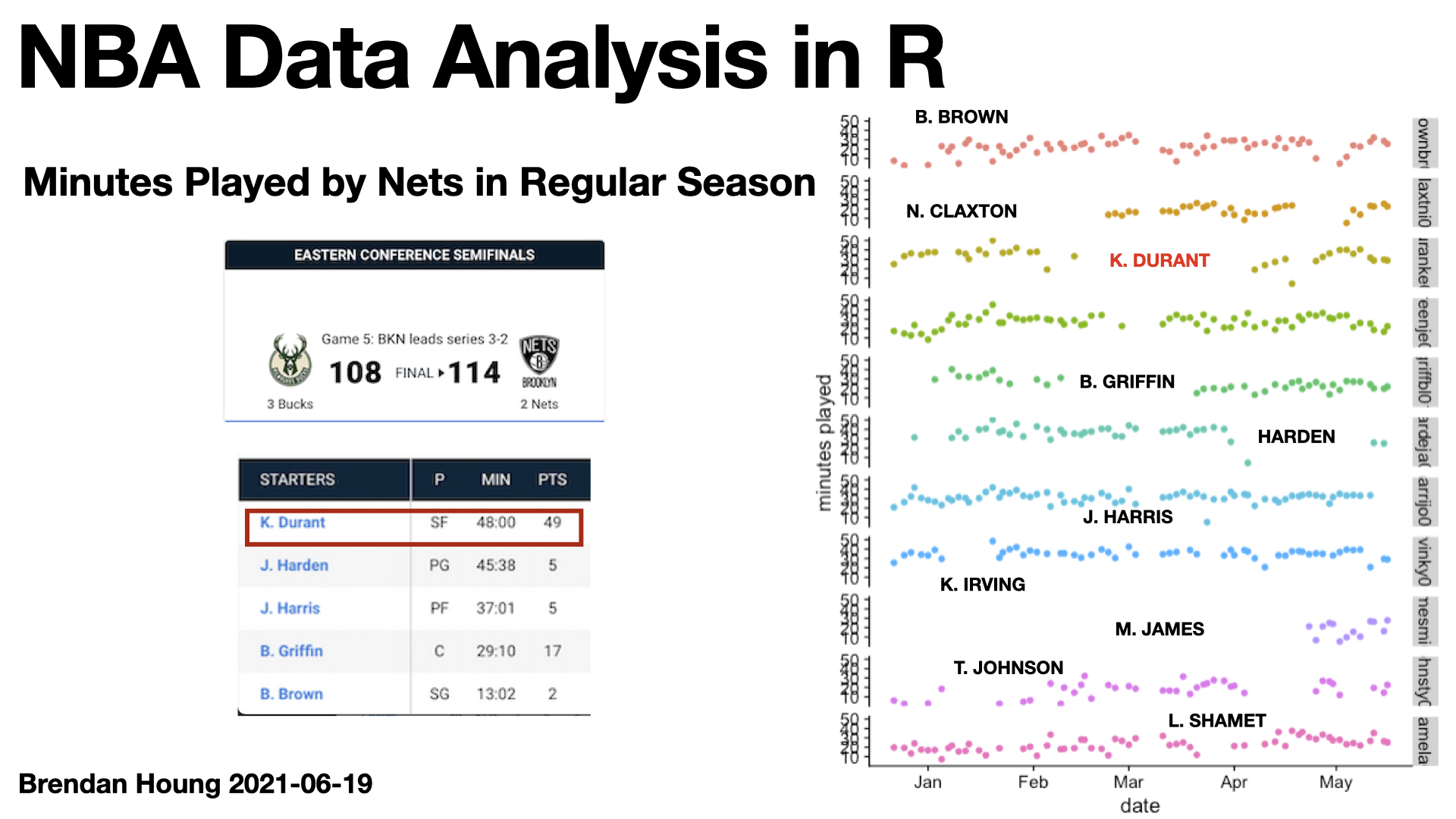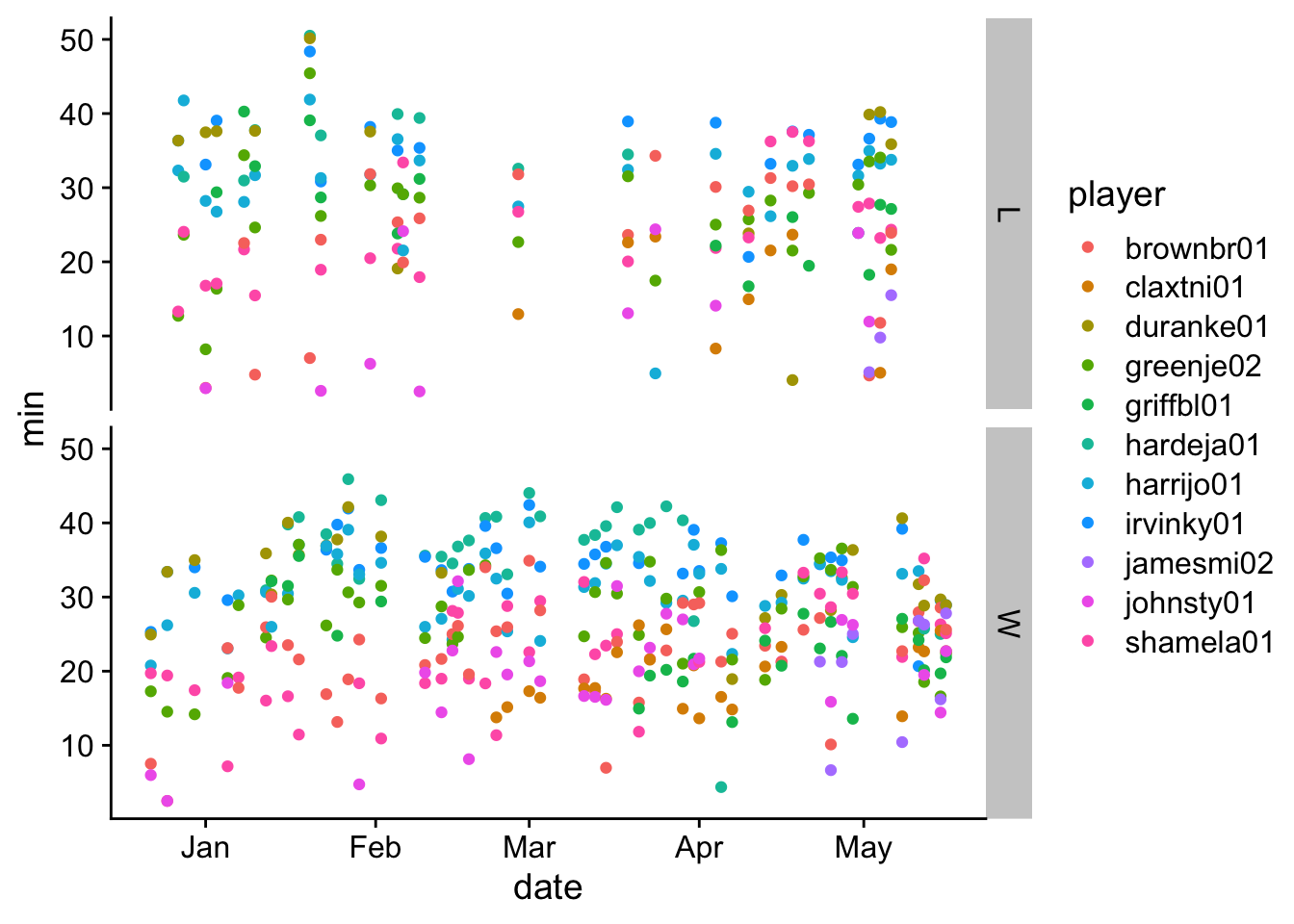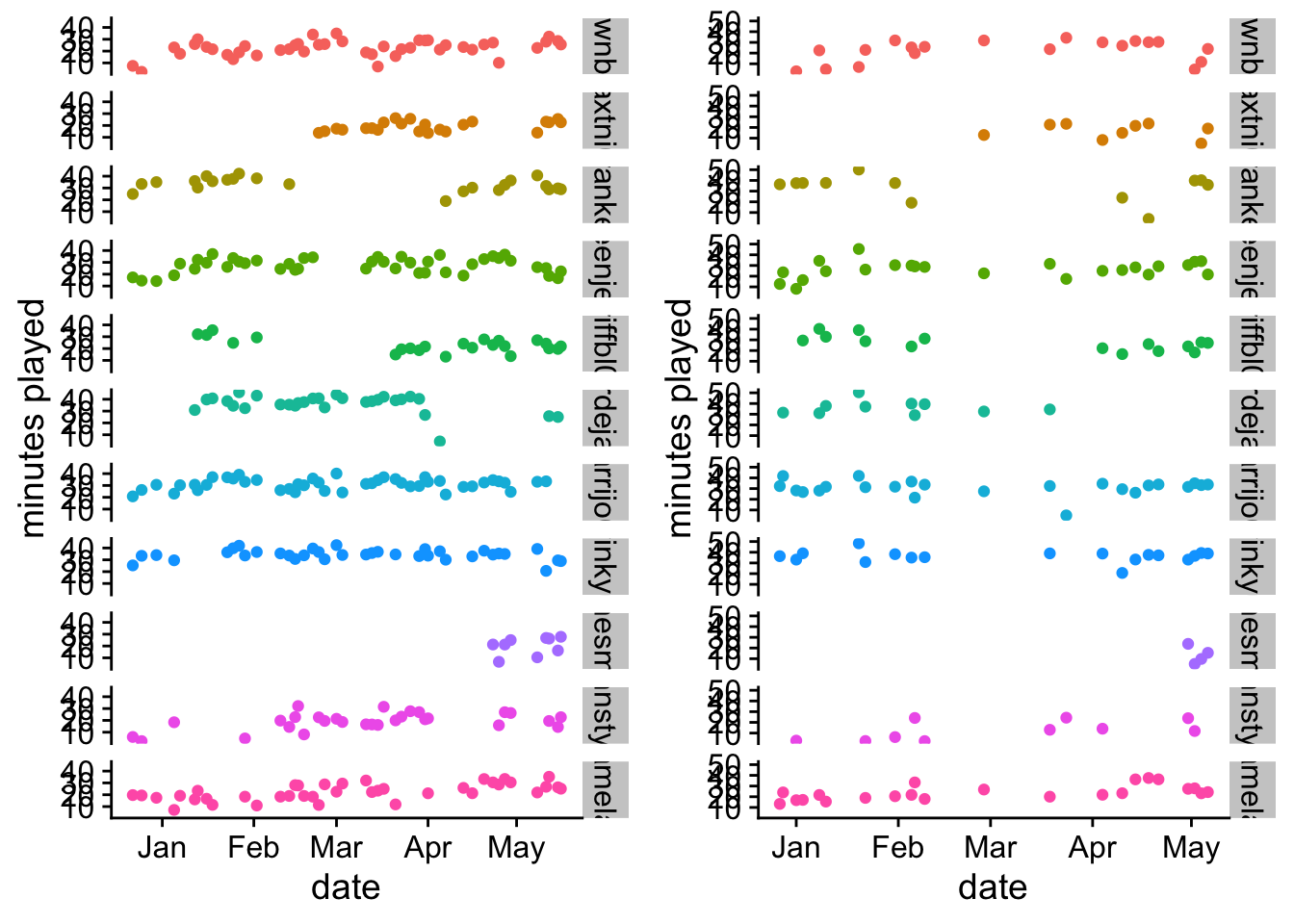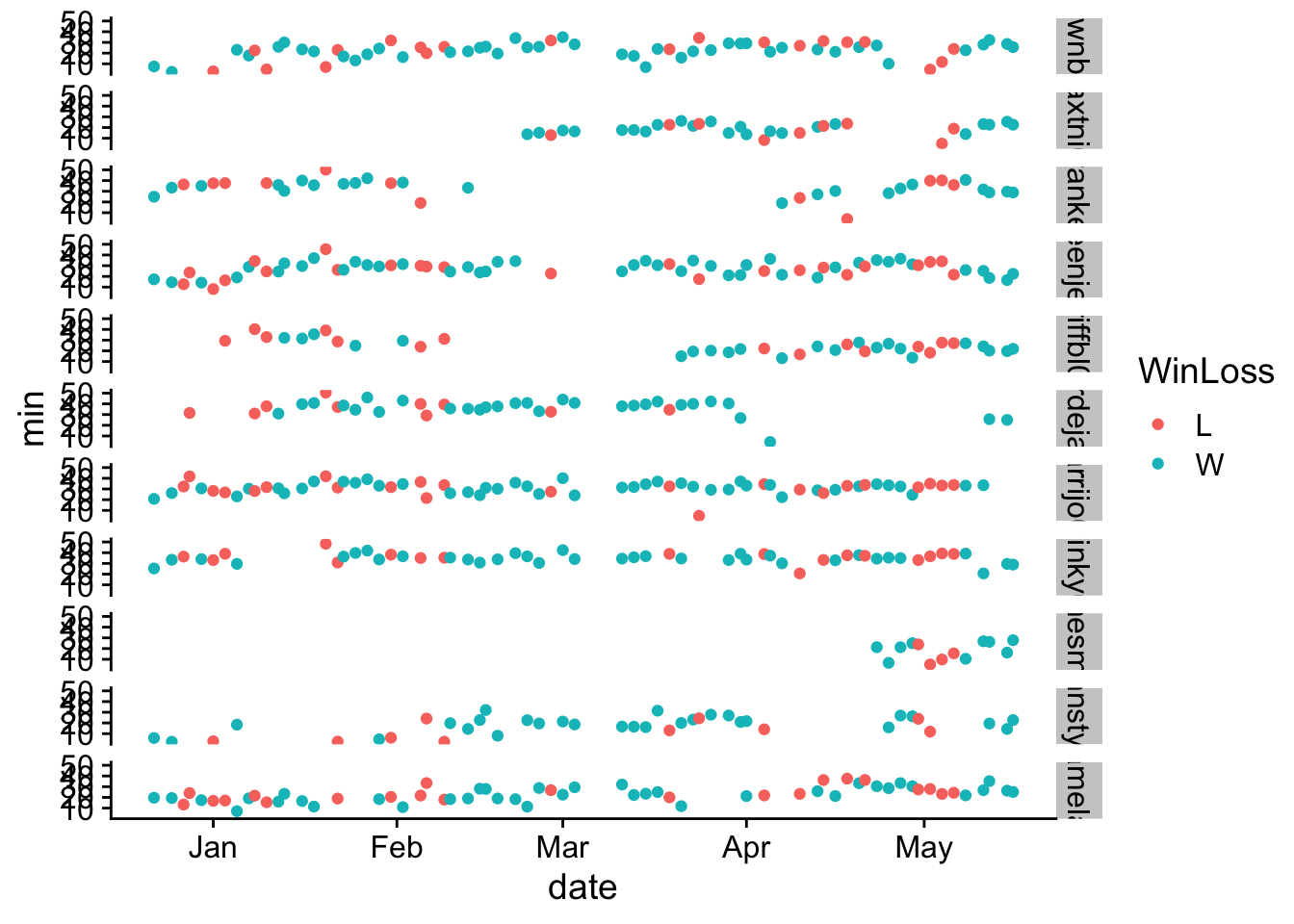Screencast #4 shows the minutes played by Nets in NBA regular season ‘21 against team win/loss and margin.``````roster <- "https://www.basketball-reference.com/teams/BRK/2021.html"

tables <- html %>% html_nodes("table") %>% html_table()
df <- tables %>% data.frame()

players <- html %>% html_nodes("table") %>% xml_find_all(".//a") %>% xml_attrs()
gamelogs <- unlist(players)
pgl <- gamelogs[grep("gamelog", gamelogs)]  %>% unique() %>% list()
pgl <- pgl[]

playoffs_pl <- pgl[c(1,2,3,4,6,9,10,14,12,15,18)]
``````
``````get_player_url <- function(path) {
}

player_urls <- unlist(lapply(FUN=get_player_url, playoffs_pl))

get_data <- function(player_url) {
tables <- html %>% html_nodes("table") %>% html_table()
df <- tables %>% data.frame()
return(df)
}
``````
``````for (p in player_urls) {
pname <- str_split(p, "/")[]
data <- get_data(p)
assign(pname, data)
}
``````
``````plist <- list()
for (p in player_urls) {
pname <- str_split(p, "/")[]
plist <- c(plist, pname)   # Add element to list
}
unlist(plist)
``````
``````##   "hardeja01" "irvinky01" "duranke01" "harrijo01" "greenje02" "shamela01"
##   "brownbr01" "claxtni01" "griffbl01" "jamesmi02" "johnsty01"
``````
``````i = 1
for (p in plist) {

if (i == 1) {
data = get(p)
data\$player <- p

} else {
df = get(p)
df\$player <- p
data = rbind(data, df)
}
i = i + 1
}
``````
``````table(data\$player)
``````
``````##
## brownbr01 claxtni01 duranke01 greenje02 griffbl01 hardeja01 harrijo01 irvinky01
##        75        75        75        75        74        71        75        75
## jamesmi02 johnsty01 shamela01
##        13        75        75
``````
``````data\$date <- as.Date(data\$Date, format="%Y-%m-%d")
dfm <- games %>% left_join(data, by=c("date"="date"))

mp <- dfm %>% select(date, MP, player, WinLoss, diff)
mp\$min <- as.difftime(mp\$MP, format = "%M:%S", units = "mins")

mp %>% filter(!is.na(player)) %>% ggplot(.) + geom_point(aes(x=date, y=min, colour=player)) +
facet_grid(WinLoss ~ .) + theme_cowplot()
````````````mp %>% filter(!is.na(player)) %>% ggplot(.) + geom_point(aes(x=date, y=min, colour=player)) +
facet_grid(player ~ .) + theme_cowplot() + labs(y="minutes played")
````````````p1 <- mp %>% filter(!is.na(player) & WinLoss == "W") %>% ggplot(.) + geom_point(aes(x=date, y=min, colour=player)) + facet_grid(player ~ .) + theme_cowplot() + theme(legend.position = "none") + labs(y="minutes played")

p2 <- mp %>% filter(!is.na(player) & WinLoss == "L") %>% ggplot(.) + geom_point(aes(x=date, y=min, colour=player)) + facet_grid(player ~ .) + theme_cowplot() + theme(legend.position = "none") + labs(y="minutes played")

plot_grid(p1, p2)
````````````mp %>% filter(!is.na(player)) %>% ggplot(.) + geom_point(aes(x=date, y=min, colour=WinLoss)) + facet_grid(player ~ .) + theme_cowplot()
``````